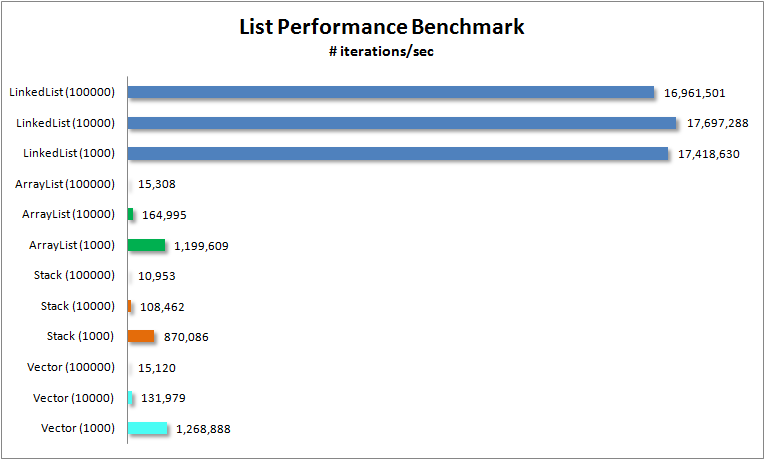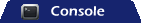# Java Collections – List Performance Measurements

I decided to put the following List-based data structures to the test in order to determine which is fastest. I tested Vector, ArrayList, LinkedList and Stack.

### Evaluating performance

I am testing how many iterations of the basic operations (add(), get(), remove()) can by performed within 1000 ms. In other words, I am testing number of operations/per second between the various collections.

```public static void testIterations(List list, int length) {
Object obj = new Object();
for (int i=0; i<length; i++) list.add(obj);
long startTime = System.currentTimeMillis();
int iterations = 0;
while (System.currentTimeMillis()-startTime < 1000) {
iterations++;
list.get(5);
list.remove(3);
}
System.out.println(list.getClass()+ " (" + length
+ "), iterations: " + iterations);
}
}```

• ArrayList
• Vector
• Stack

### List Performance Benchmark### Full Program Listing

```package com.avaldes.tutorials;

import java.util.ArrayList;
import java.util.List;
import java.util.Stack;
import java.util.Vector;

public class ListPerformanceBenchmark {
final static int SMALL  = 1000;
final static int MEDIUM = 10000;
final static int LARGE  = 100000;

public static void testIterations(List list, int length) {
Object obj = new Object();
for (int i=0; i<length; i++) list.add(obj);
long startTime = System.currentTimeMillis();
int iterations = 0;
while (System.currentTimeMillis()-startTime < 1000) {
iterations++;
list.get(5);
list.remove(3);
}
System.out.println(list.getClass()+ " (" + length+ "), iterations: "
+ iterations);
}

public static void testStackIterations(Stack list, int length) {
Object obj = new Object();
for (int i=0; i<length; i++) list.add(obj);
long startTime = System.currentTimeMillis();
int iterations = 0;
while (System.currentTimeMillis()-startTime < 1000) {
iterations++;
list.push(obj);
list.search(5);
list.pop();
}
System.out.println(list.getClass()+ " (" + length+ "), iterations: "
+ iterations);
}

public static void main(String[] args) {

testIterations(new Vector(), SMALL);
testIterations(new Vector(), MEDIUM);
testIterations(new Vector(), LARGE);

testStackIterations(new Stack(), SMALL);
testStackIterations(new Stack(), MEDIUM);
testStackIterations(new Stack(), LARGE);

testIterations(new ArrayList(), SMALL);
testIterations(new ArrayList(), MEDIUM);
testIterations(new ArrayList(), LARGE);

}
}
```

### Output```class java.util.Vector (1000), iterations: 1483581
class java.util.Vector (10000), iterations: 149238
class java.util.Vector (100000), iterations: 15220
class java.util.Stack (1000), iterations: 1315147
class java.util.Stack (10000), iterations: 131550
class java.util.Stack (100000), iterations: 15348
class java.util.ArrayList (1000), iterations: 1197541
class java.util.ArrayList (10000), iterations: 162981
class java.util.ArrayList (100000), iterations: 15288